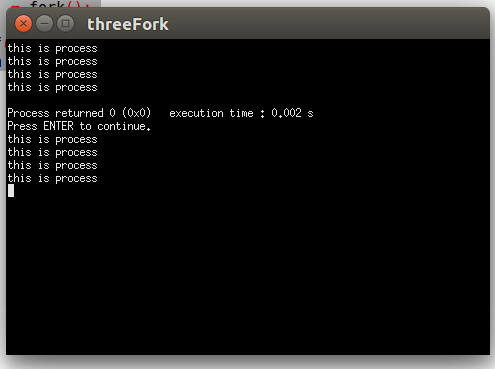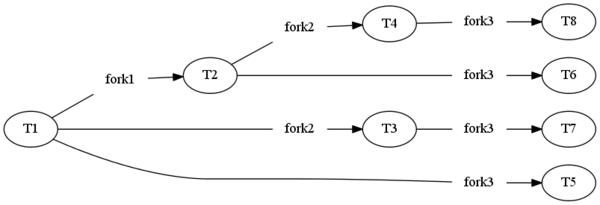# linux下连续三次fork() --深度理解进程创建函数

#include<stdio.h>
#include<unistd.h>
int main()
{
pid_t pid;
int count = 0;
pid = fork();   //fork一个进程
if(pid == 0)
{               //pid为0,
printf("this is child process, pid is %d\n",getpid());//getpid返回的是当前进程的PID
count+=2;
printf("count = %d\n",count);
}
else if(pid > 0)
{
printf("this is father process, pid is %d\n",getpid());
count++;
printf("count = %d\n",count);
}
else
{
fprintf(stderr,"ERROR:fork() failed!\n");
}
return 0;
}


fork的时候发生什么？
①执行到这一句的时候，一个进程被创建了，这个进程与父进程一样，拥有一套与父进程相同的变量，相同的一套代码，这里可以粗浅的理解为子进程又复制了一份main函数。这里返回一个子进程的进程号，大于0。（第一次fork）

②子进程怎么执行：

#include<stdio.h>
#include<unistd.h>
int main()
{
pid_t pid;
int count = 0;
pid = fork();
pid = fork();
pid = fork();

printf("this is process\n");

return 0;
}#include <stdio.h>
#include <stdlib.h>
#include <sys/unistd.h>

int main(int argc, char *argv[])  {
int i,j,status;
int pid;

for(i=0; i<3;i++){
if((pid[i]=fork()) >0){

printf("This is child process pid=%d\n",pid[i]);

} else{
printf("This is father process pid=%d\n",pid[i]);
exit( EXIT_SUCCESS);
}

}

return EXIT_SUCCESS;
}